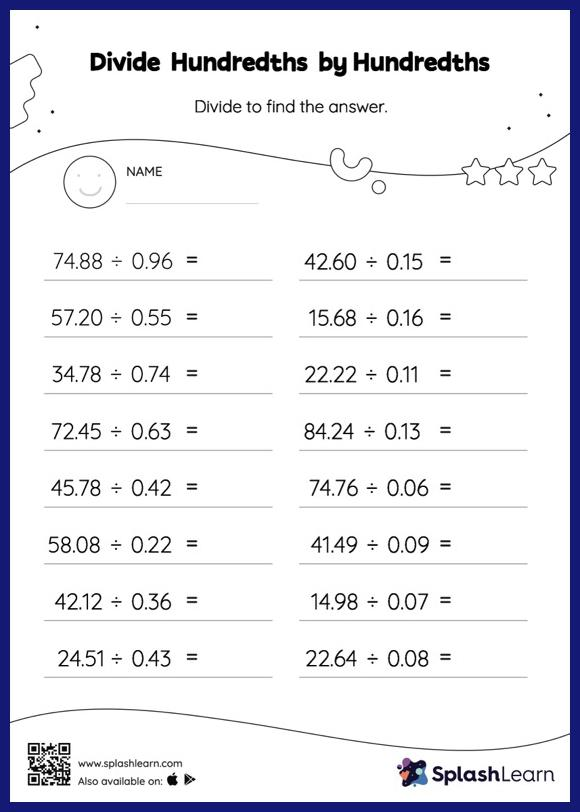# Divide Hundredths by Hundredths without Remainder: Horizontal Division Worksheet

Home > Divide Hundredths by Hundredths without Remainder: Horizontal DivisionStudents first divide as though there is no decimal when dividing two decimals. They then add a decimal point at the correct location in the quotient depending on the number of decimal digits in each number. This divide hundredths by hundredths without remainder worksheet gives students a thorough practice of this concept. As the worksheet uses a horizontal format, it allows for more creativity when it comes to how the student solves the problem. In contrast, in the vertical format, students usually employ the standard method to solve.